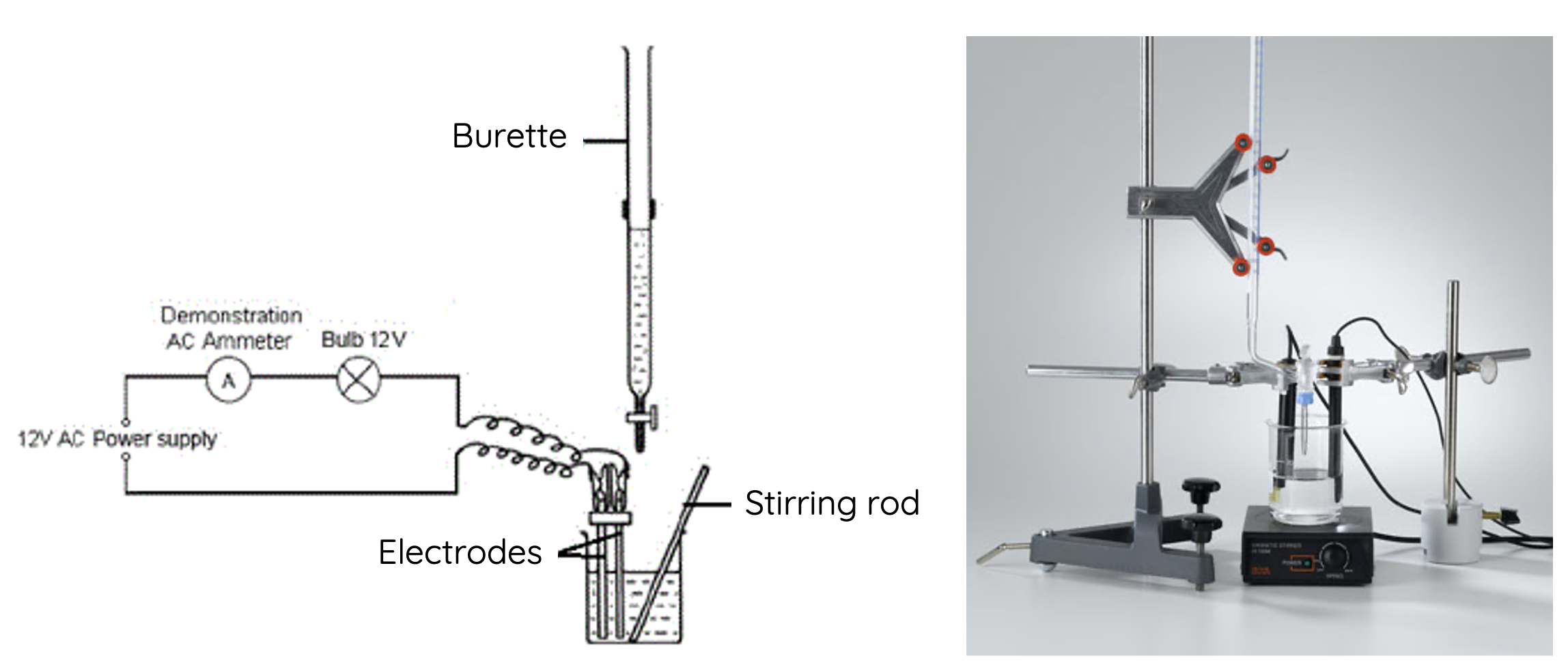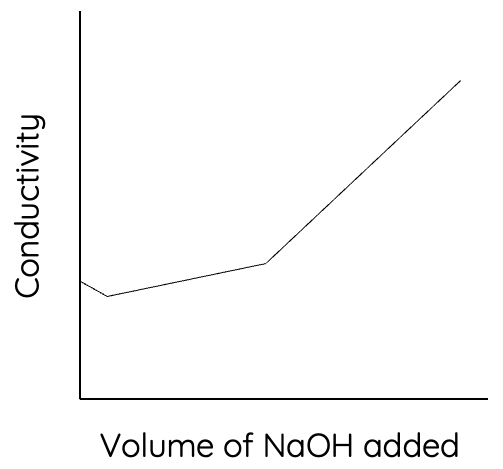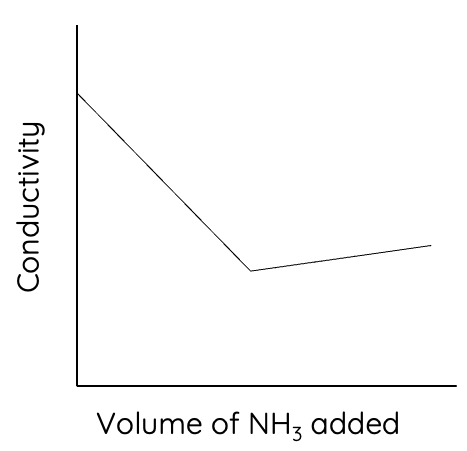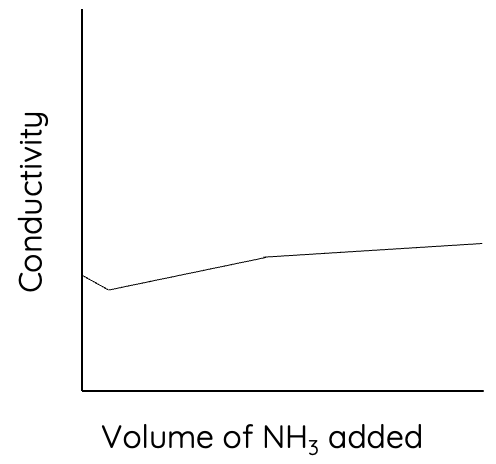# HSC Chemistry – Conductometric Titration

This is part of the HSC Chemistry course under the topic Quantitative Analysis.

### HSC Chemistry Syllabus

• investigate titration curves and conductivity graphs to analyse data to indicate characteristic reaction profiles, for example:
– strong acid/strong base
– strong acid/weak base
– weak acid/strong base (ACSCH080, ACSCH102)

### Conductometric Titration

This video discusses the principles of conductometric titration. The video also explains the conductivity graphs of neutralisation reactions betwee

• strong acid and strong base

• strong acid and weak base

• weak acid and strong base

• weak acid and weak base

### Conductivity of Acid and Base Solutions

An ionic solution's conductivity is affected by the presence of its ions as they act as charge (electron) carriers.

Arrhenius acids dissociate and produce hydrogen ions in water. Brønsted-Lowry acids donate protons to water to form hydronium ions. The conjugate base of an acid can also be an ion.
$$HCl(aq) \rightarrow H^+(aq) + Cl^–(aq)$$

Arrhenius bases dissociate and produce hydroxide ions in water. Brønsted-Lowry bases accept protons from water to form hydroxide ions. The conjugate acid of a base can also be an ion.
$$NaOH(aq) \rightarrow Na^+(aq) + OH^–(aq)$$

An acid or base solution's conductivity depends on two main factors:
• Concentration: greater concentration leads to greater conductivity
• Strength: stronger acids/bases means there is a greater degree of ionisation which leads to a higher concentration of ions. Therefore, stronger acids and bases produce more conductive solutions.

### Conductivity of Ions

Some ions are better conductors or charge carriers than others. Hydrogen and hydroxide ions are the most conductive due to their smaller sizes.### How does Conductometric Titration Work?In a conductometric titration, the progress of titration is measured using a conductivity meter.

A conductivity meter measures the conductance of the solution by passing an electric current through the electrodes in the beaker. A solution with higher conductivity will yield a higher current reading on the conductivity meter.

As the concentrations of ions change throughout titration, the conductivity of the solution changes. Conductometric titration determines the equivalence point based on a turning point in the change of conductivity. This 'turning point' has a different characteristic on the graph depending on the type of acid and base used.

### Strong Acid and Strong Base Conductometric Titration

Suppose hydrochloric acid is added from the burette into a beaker containing sodium hydroxide.

$$HCl(aq) + NaOH(aq) \rightarrow NaCl(aq) + H_2O(l)$$The conductivity of the solution is initially relatively high because a strong base e.g. NaOH completely dissociates to produce hydroxide ions.

As HCl is added, the concentration of hydroxide ions decreases due to the neutralisation between NaOH and HCl.

Although the the concentration of chloride ions increases with titration, the conductivity still decreases because it is outweighed by the loss of hydroxide ions as they are more conductive.

When the titration reaches the equivalence point, the conductivity of the solution reaches a minimum value as hydroxide ions are completely neutralised by the addition of HCl.

After the equivalence point, the conductivity of the solution increases due to increasing concentration of hydrogen ions.

### Weak Acid and Strong Base Conductometric Titration

Suppose sodium hydroxide is added from the burette into a beaker containing acetic acid.

$$NaOH(aq) + CH_3COOH(aq) \rightarrow NaCH_3COO(aq) + H_2O(l)$$The conductivity of the solution is initially relatively low because acetic acid (weak acid) partially dissociates to produce a relatively small amount of hydrogen ions and its conjugate base, acetate ions.

As NaOH is added, it neutralises the small number of hydrogen ions present in solution, leading to a small reduction in conductivity.

After the initial number of hydrogen ions are neutralised, the equilibrium position of acetic acid's ionisation shifts to the right to produce more hydrogen ions and acetate ions (Le Chatelier's principle).

$$CH_3COOH(aq) \rightleftharpoons H^+(aq) + CH_3COO^–(aq)$$

The newly produced hydrogen ions are quickly neutralised by the addition of NaOH. However, the conductivity of the solution increases due to a greater concentration of acetate ions and sodium ions.

After the equivalence point, the conductivity of the solution increases at a faster rate. This is due to the increase in concentration of sodium ions, acetate ions, and most importantly hydroxide ions.

### Strong Acid and Weak Base Conductometric Titration

Suppose that ammonia solution is added from the burette into a beaker containing hydrochloric acid.

$$NH_3(aq) + HCl(aq) \rightarrow NH_4Cl(aq)$$The initial conductivity of the solution is relatively high because HCl is a strong acid that completely dissociates to produce a relatively large concentration of hydrogen ions and chloride ions.

As ammonia is added, concentration of hydrogen ions decreases, causing the conductivity to fall.

At the equivalence point, the conductivity reaches its lowest value because all hydrogen ions have been neutralised by the addition of ammonia.

After the equivalence point, the addition of excess ammonia only increases the conductivity slightly. This is because ammonia a weak base that partially ionises in water to produce a relatively small concentration of ammonium and hydroxide ions

$$NH_3(aq) + H_2O(l) \rightleftharpoons NH_4^+(aq) + OH^–(aq)$$

### Weak Acid and Weak Base Conductometric Titration (Extension)

Suppose ammonia is added from the burette into a beaker containing acetic acid.

$$NH_3(aq) + CH_3COOH(aq) \rightleftharpoons NH_4CH_3COO(aq)$$The conductivity is initially relatively low because acetic acid is a weak acid that partially dissociates to produce a relatively small concentration of hydrogen and acetate ions.

As ammonia added, it neutralises with the small number of hydrogen ions, causing the conductivity to decrease slightly.

By Le Chatelier's principle, the equilibrium position of acetic acid's ionisation in water shifts to the right to produce more hydrogen ions and acetate ions. This leads to an increase in conductivity.

$$CH_3COOH(aq) \rightleftharpoons H^+(aq) + CH_3COO^–(aq)$$

After the equivalence point, the conductivity increases at a faster rate because ammonia ionises to form hydroxide ions and ammonium ions. The increase in concentration of these ions contribute to a greater conductivity.